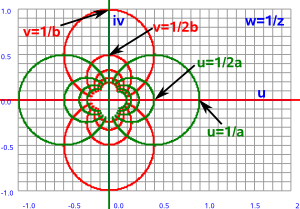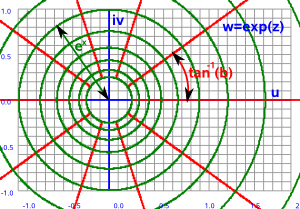# Maths - Functions Of A Complex Variable

On this page we discuss how to extend the scalar functions (that we discussed on this page) to the situation where the domain and/or codomain are complex numbers, we also discuss functions like normalise which are specific to complex numbers.

We can categorise these functions according to the the following properties:

• Conformal - preserves angles
• Anticonformal - reverses angles
• Non-conformal - does not conserve angles
preservation of angles preservation of distances Analytic
Conjugate Anticonformal
Powers of complex numbers
Exponential Function     Riemann surface
Inverse Function Conformal   yes, except at 0 and &in
Reflection in circle Anticonformal
Norm (normalise)
Möbius transformation

## Evaluating Complex Functions

We can evaluate the value of many complex functions by using infinite series in the same way that we can for scalar functions. We just use the same series that we would use for scalar functions but plug in complex values instead of real values. So if a series exists for real values we can evaluate the complex function. For example:

 ln(1+z) z - z 2/2! + z 3/3! ... +(-1)r+1zr/(r)! -1 < z <= 1 exp(z) 1 + z 1/1! + z 2/2! + z 3/3! ... + zr/(r)! all values of z

Alternatively we can derive the real and imaginary parts in terms of the real functions:

function of complex variable real and imaginary terms
exp(x+i y) exp(x)*(cos(y)+i sin(y))
ln(x+i y) 0.5*ln(x²+y²) + i atan(x/y)
sin(x+i y)

cosh(-y) sin(x) - i sinh(-y) cos(x)

cos(x+i y)

cosh(-y) cos(x) + i sinh(-y) sin(x)

tan(x+i y)

(tan(x) -i tanh(-y)) /
(1 +i tanh(-y) tan(x))

sinh(x+i y) sinh(x) cos(y) + i cosh(x) sin(y)
cosh(x+i y) cosh(x) cos(y) + i sinh(x) sin(y)
tanh(x+i y) (tanh(x) + i tan(y)) /
(1 +i tanh(x) tan(y))

We can convert between the circular and hyperbolic functions as follows:

• sin(z) = -i sinh(iz)
• cos(z) = -cosh(iz)
• tan(z) = -i tanh(iz)

## Conjugate

When we multiply a complex number by its conjugate we get a real number, in other words the imaginary part cancels out.

## Inverse Function

To calculate the inverse value (1/z) we multiply the top and bottom by the conjugate which makes the denominator a real number.

z plane   w plane-->

w=1/zhow this plot was produced.

Let the components of the input and output planes be:

z = x + i y and w = u + i v

In this case w = 1/z

so:

w = 1/(x + i y)

As usual, we evaluate the inverse by multiplying top and bottom by the conjugate:

w = (x - i y)/(x + i y)(x - i y)

w = (x - i y)/(x² + y²)

so the u and v components are:

u = x /(x²+y²)
v = -y /(x²+y²)

This function has some very interesting and useful properties:

• Straight lines through the origin map to themselves.
• Straight lines not through the origin map to circles.
• Circles through the origin map to straight lines.
• Circles not through the origin map to circles.
• Applying the inverse function twice restores the original.
• The inverse function can be decomposed into a conjugate and a reflection in a circle.

So this function can be used to map between circles and lines. We can also extend the concept to 3 dimensions which allows us to map the surface of a sphere to the plane (see stereographic projections).

### Möbius Transform

This can be generalised to the Möbius Transform (described here):Where:

• z = input (complex values)
• w = output (complex values)
• a,b,c,d = parameters (complex values)

The inverse function is a special case where a=d=0 and b=c=1 we get:

w=1/z

If we now allow d to be non-zero we can see that this will just shift the line in the z-plane:

w=1/(z+d)

If we now allow c to scale we can see that this will scale the position on the line in the z-plane:

w=1/(cz+d)

If we now allow a and b to vary things get a bit more complicated but we can see that we can move the circle around the w plane.

## Exponential Function

There are two methods to calculate the exponential function:

z plane   w plane-->

w=ezhow this plot was produced.

Let the components of the input and output planes be:

z = x + i y and w = u + i v

In this case w = ez

so:

w = e(x + i y)

by the rules of exponents:

w = exei y

applying Euler's equation we get:

w = ex(cos(y) + i sin(y))

so the u and v components are:

u = excos(y)
v = exsin(y)

## Log Function

The natural log, that is the log to the base e, is the inverse of the exponetial function. So if,

w = ln(z)

then taking the exponent of each side gives,

ew = z

so we have

x = eucos(v)
y = eusin(v)

which gives:

u = ln(√(x² + y²))
v = atan(x/y)

## Powers of complex numbers

Here is the plot for (x + i y)² the more general expression for (x + i y)n is given on this page.

z plane   w plane-->

w=z²how this plot was produced.

Pure real values always square to a positive value and pure imaginary values always square to a negative value. However real and imaginary parts together cover the whole plane.

Let the components of the input and output planes be:

z = x + i y and w = u + i v

lets take the example of the square function w = z²

so:

w = (x + i y)²

multiplying out gives:

w = x² - y² + i 2 x y

so the u and v components are:

u = x² - y²
v = 2 x y

## Norm

This is the distance (r) of a + i b from the origin.

It is written as:

r = | a + i b |

by Pythagoras:

r = | a + i b | = math.sqrt(a*a + b*b)

Check that:

|a + i b|*|c + i d| = |a*c - b*d + i (a*d + b*c)|

## Möbius transformation

The mapping between the surface of the sphere and the plane can be represented by the Möbius transformation of the form:

 M(z)= az + b cz + d

Where:

• z = complex variable
• a,b,c & d = complex constants

## Further Study

I think its interesting to compare these graphs for complex numbers to the equivalent graphs for double numbers and dual numbers.Visual Complex Analysis - If you already know the basics of complex numbers but want to get an in depth understanding using an geometric and intuitive approach then this is a very good book. The book explains how to represent complex transformations such as the Möbius transformations. It also shows how complex functions can be differentiated and integrated.